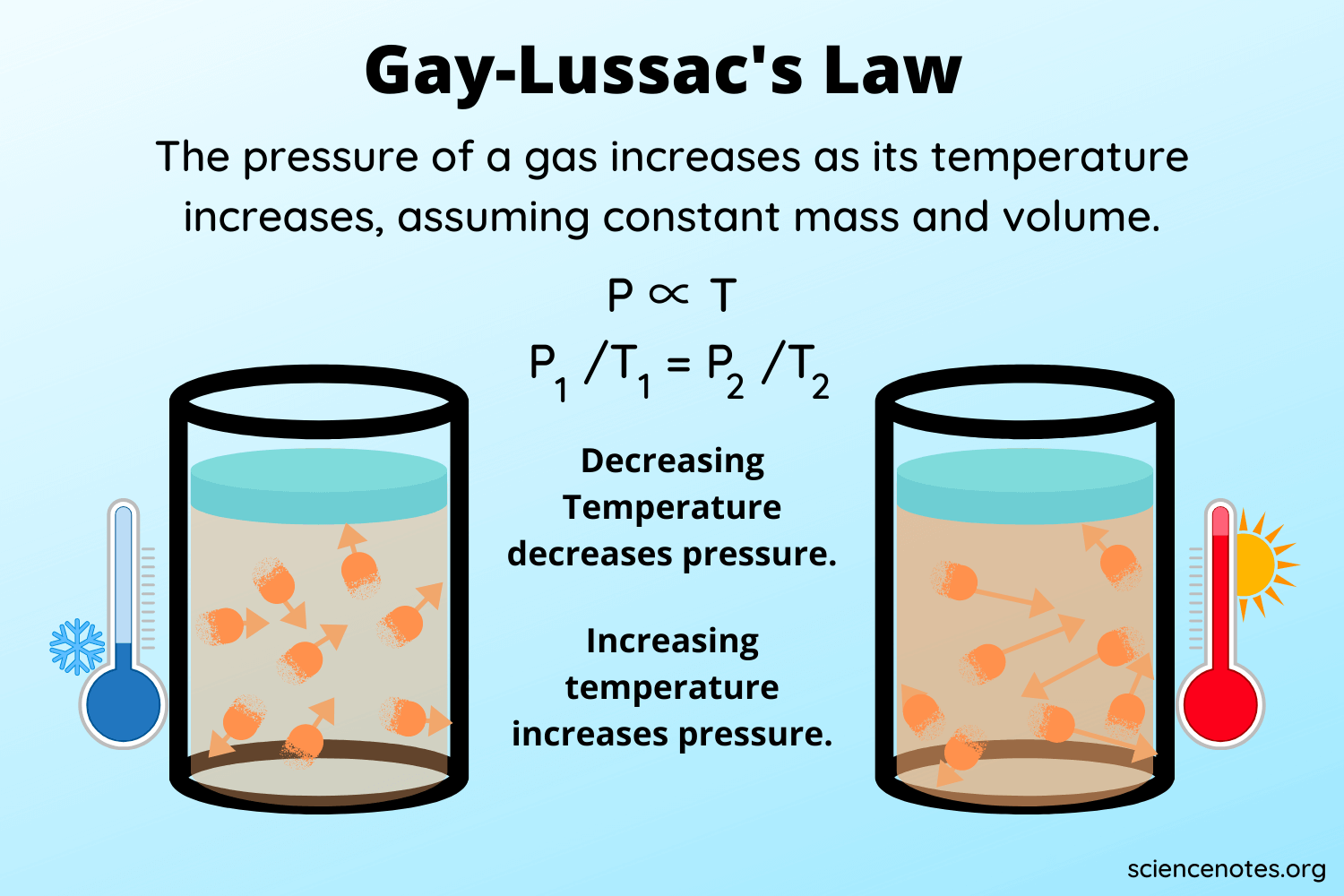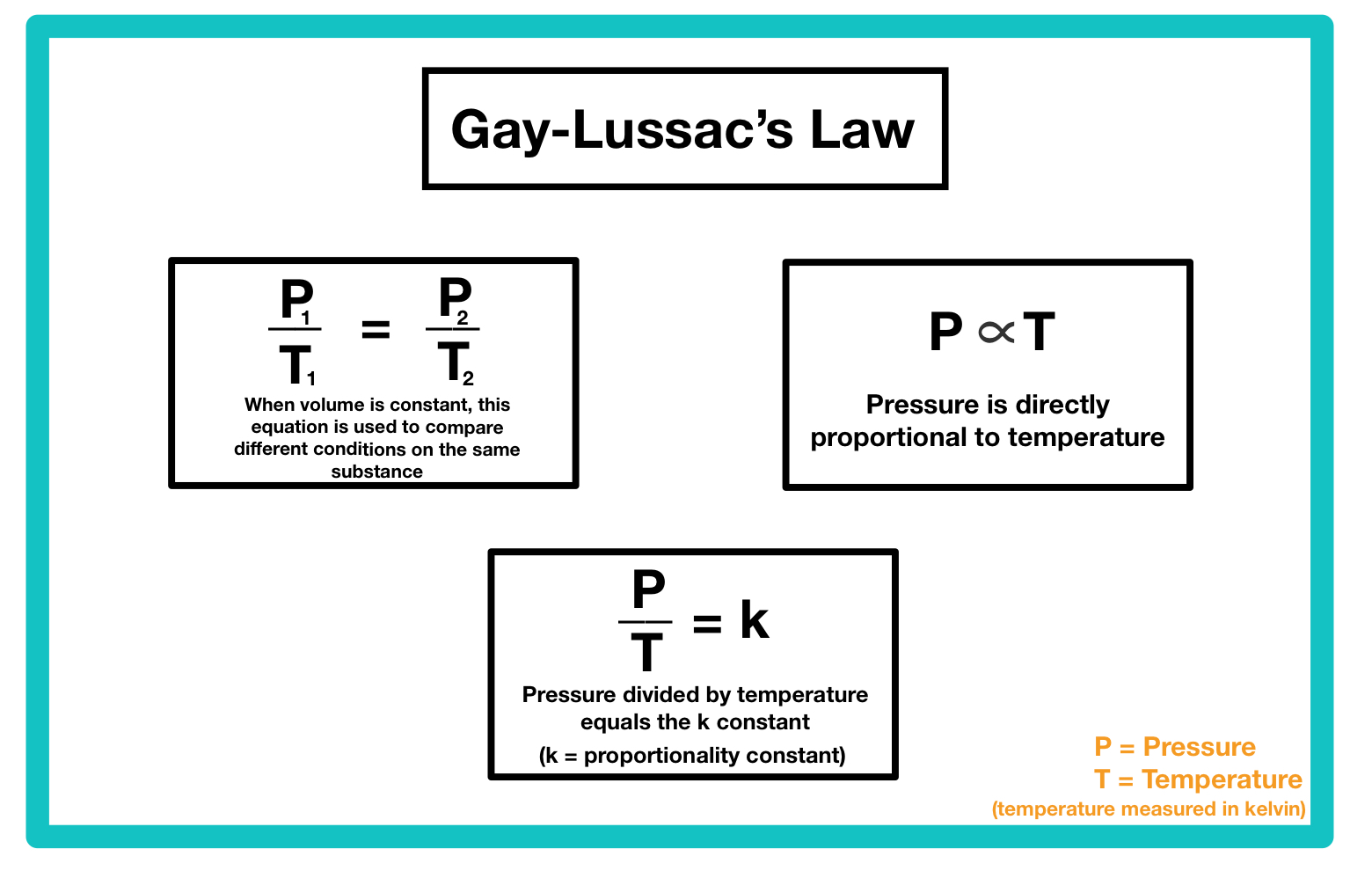Sarah Taylor

HS-PS1-6

# Gay-Lussac’s Law of Ideal Gasses Study Guide

🗻 Big Picture: Gay-Lussac’s law states that the pressure of a gas is directly proportional to the absolute temperature of the gas at a constant volume and amount of gas.

### Lesson Objectives:

• Define Gay-Lussac’s law
• Describe the relationship between the pressure and temperature of gas at a constant volume and amount of gas.
• Solve problems using the Gay-Lussac’s law equation

## IntroductionSource

Imagine it’s a hot summer day and you’re at the beach with your friends. To protect yourself from the harmful UV rays from the sun, you bring a can of aerosol sunscreen. While you play in the water, the heat from the surrounding environment begins to slowly raise the temperature inside the can higher and higher. When you come back from the water to reapply the sunscreen, you find that the can has exploded all over your belongings. This unfortunate incident can be explained by Gay-Lussac’s law. Let’s talk about how.

### Gay-Lussac’s Law Definition, Formula, & DerivationSource

Gay-Lussac’s law states that the pressure of a gas is directly proportional to the absolute temperature of the gas at a constant volume and amount of gas. Mathematically, we can express this as:

P ∝ T

Meaning that pressure is proportional to temperature. Because Gay-Lussac’s law states that volume and the amount of gas remain constant, we can replace them with the constant k to translate it into the algebraic expression:

P/T = k

Where:

• P = pressure
• T = absolute temperature

If we let the initial pressure equal:

P1/T2 = k

and the final volume equal:

P2/T2 = k

We can combine these equations to form the Gay-Lussac’s law equation:

P1/T1 = P2/T2

Where:

• P1 = initial pressure
• T1 = initial absolute temperature
• P2 = final pressure
• T2 = final absolute temperature

### Sample Problem: Gay-Lussac’s Law

A sample of boron gas inside a rigid container at 18°C is heated to a temperature of 43°C. The initial pressure of the container was 4.3 atm. Given this information, what is the final pressure of the boron gas?

Step 1: Define the variables

P1 = 4.3 atm

P2 = ?

T1 = 18°Cl = 291.15 K

T2 = 43°C = 316.15 K

Step 2: Set up the equation

P1/T1 = P2/T2

4.3 atm/291.15 K = P2/316.15K

Step 3: Calculate

4.3 atm/291.15 K = P2/316.15K

P2 = (4.3 atm/316.15K) • 291.15 K

P2 = 1359.445 atm/K • 291.15 K

P2 = 4.669 atm

## 💡 Summary

• Gay-Lussac’s law states that the pressure of a gas is directly proportional to the absolute temperature of the gas at a constant volume and amount of gas.
• The Avogadro’s law equation is P1/T1 = P2/T2.

### 🧐 FAQs

1. What is Gay-Lussac’s law?

Gay-Lussac’s law states that the pressure of a gas is directly proportional to the absolute temperature of the gas at a constant volume and amount of gas.

2. What is the formula used to represent Gay-Lussac’s law?

The mathematical expression for Boyle’s law formula is: P1/T1 = P2/T2.

We hope you enjoyed studying this lesson and learned something cool about Gay-Lussac’s Law Of Ideal Gasses! Join our Discord community to get any questions you may have answered and to engage with other students just like you! Don't forget to download our App to experience our fun, VR classrooms - we promise, it makes studying much more fun! 😎

## Sources

1. Admin. “Gay-Lussac’s Law - Statement, Formula, Detailed Explanation.” BYJUS, BYJU’S, 4 Jan. 2016, https://byjus.com/chemistry/gay-lussacs-law/.
2. CK-12 Foundation. “CK12-Foundation.” CK-12 Foundation, CK-12, https://flexbooks.ck12.org/cbook/ck-12-chemistry-flexbook-2.0/section/14.5/primary/lesson/gay-lussacs-law-chem/. Accessed 19 Apr. 2022.
3. “Gay-Lussac’s Law Lesson Plan | Study.Com.” Study.Com, Study.Com, https://study.com/academy/lesson/gay-lussacs-law-lesson-plan.html. Accessed 19 Apr. 2022.